5.3. Heating and cooling of the intracluster gas

In this section, processes that heat or cool the intracluster gas are reviewed. Only processes that affect the total energy of the gas are considered here, while processes (such as heat conduction or mixing) that redistribute the gas energy are discussed in Section 5.4.

The primary cooling process for intracluster gas is the emission of radiation by the processes discussed in Section 5.2.2 above. At temperatures Tg3 × 107 K, the main emission mechanism is thermal bremsstrahlung, for which the total emissivity is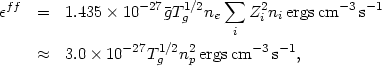(5.21)

where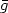is the integrated Gaunt factor, and zi and ni are the charge and number density of various ions i. The second equation follows from assuming solar abundances and= 1.1 in a fully ionized plasma. For Tg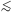3 × 107 K, line cooling becomes very important. Raymond et al. (1976) give the cooling rate at lower temperatures; a very crude approximation is (McKee and Cowie, 1977)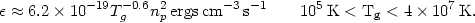(5.22)

In assessing the role of cooling in the intracluster gas, it is useful to define a cooling time scale as tcool(d ln Tg / dt)-1. For the temperatures that apply for the intracluster gas in most clusters, equation (5.21) gives a reasonable approximation to the X-ray emission. If the gas cools isobarically, the cooling time is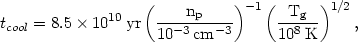(5.23)

which is longer in most clusters than the Hubble time (age of the universe). Thus cooling is not very important in these cases. However, at the centers of some clusters the cooling time is shorter than the Hubble time, and these clusters are believed to have cooling flows (Section 5.7).